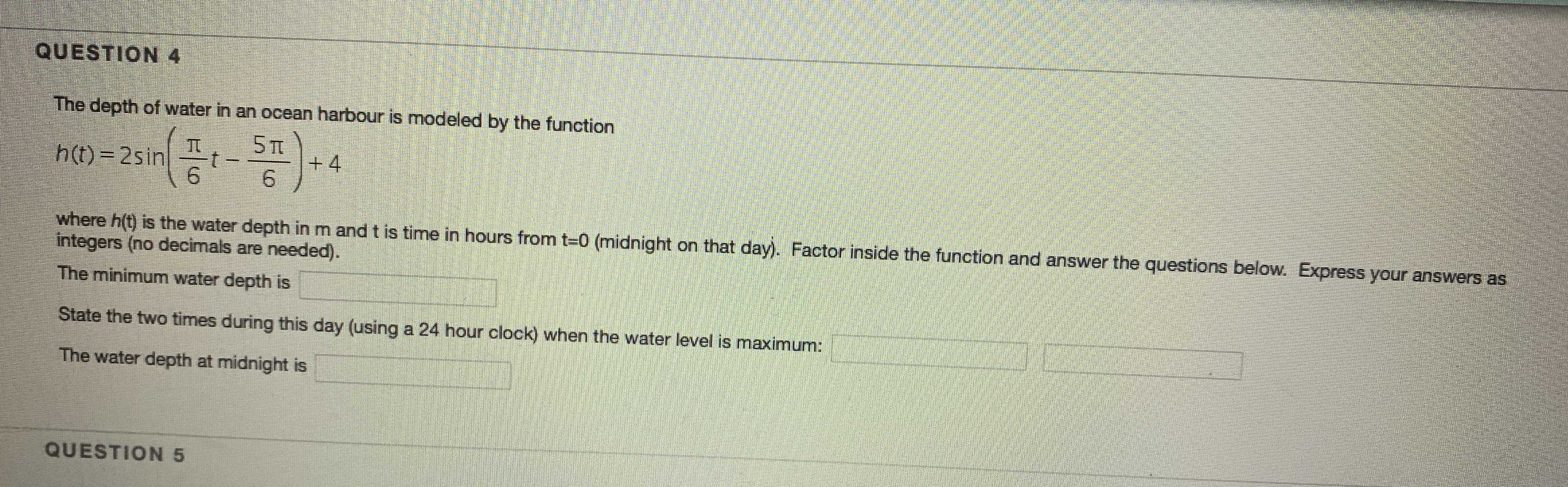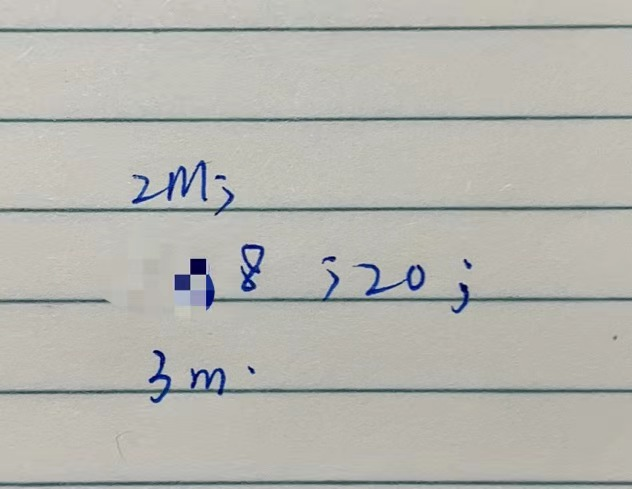### Still have math questions?

Algebra
QuestionQUESTION $$4$$ The depth of water in an ocean harbour is modeled by the function

$$h ( t ) = 2 \sin ( \frac { \pi } { 6 } t - \frac { 5 \pi } { 6 } ) + 4$$ where $$h ( t )$$ is the water depth in $$m$$ and $$t$$ is time in hours from $$t = 0$$ (midnight on that day). Factor inside the function and answer the questions below. Express your answers as integers (no decimals are needed). The minimum water depth is State the two times during this day (using a $$24$$ hour clock) when the water level is maximum: The water depth at midnight is